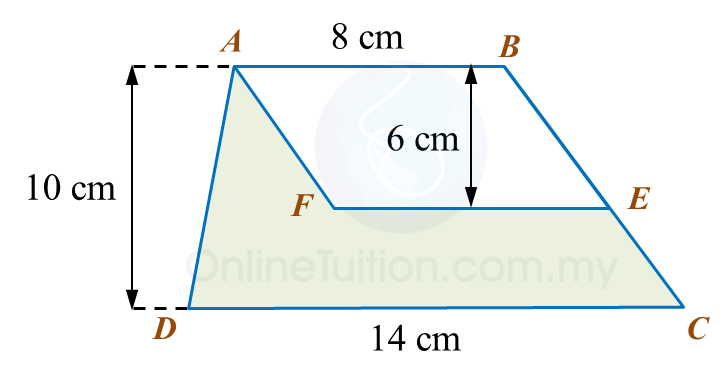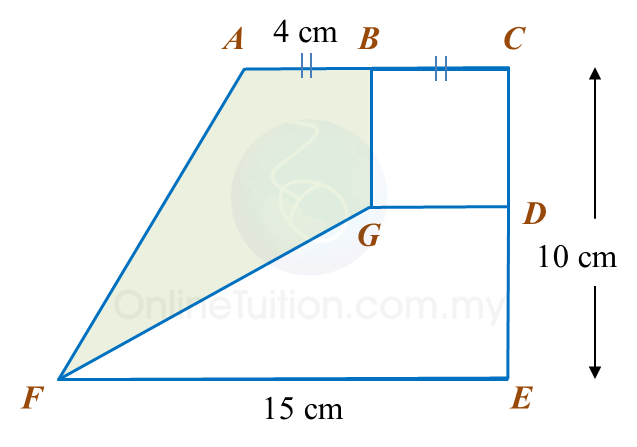# 10.2.1 Perimeter and Area, PT3 Practice

Question 1:
In the diagram, ABCD is a trapezium and ABEF is a parallelogram.Calculate the area, in cm2, of the coloured region.

Solution
:

Question 2:
Diagram below shows a rectangle ABCD.Calculate the area, in cm2, of the coloured region.

Solution:

 
     
Question 3:
 In diagram below, ACEF is a trapezium and BCDG is a square.Calculate the area, in cm2, of the coloured region.
 
 (adsbygoogle = window.adsbygoogle || []).push({}); Solution: